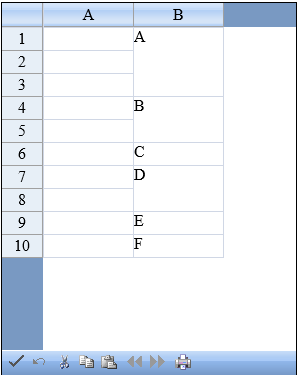﻿ Cell Merging on Client-side in Spread# Cell Merging on Client-side in Spread

Recently one of our customers had a requirement of merging cells with same values i.e. contiguous rows in one column having the same value should be displayed as a single cell. Spread for ASP.Net currently supports creating span of cells from the server side. Spanning of cells simply displays the value of first cell in the spanned cell range, as the value of the spanned cell. This blog describes how we can use the rowSpan and colSpan properties of Spread cell on the client side to merge the cells with the same value. Here is the simple javascript function that loop through the rows to find the group of consecutive rows having same value in a specific column. Once we get this cell range we simply set the rowSpan property of the first cell equal to the number of rows in this cell range. This would create a spanned cell, however it appears as if the cells with the same value have been merged. Here is the code:

``````
<script type="text/javascript">
function Button2_onclick()
{
var r = 0;
while (r != rc - 1)
{
r1 = r;
var inc = 0;
while (r1 != -1)
{
var val2 = spread.GetValue(r1 + 1, 1);
if (val1 == val2)
{
inc++;
r1++;
}
else
{
cell.rowSpan = inc + 1;
r = r1 + 1;
r1 = -1;
}
}
}
Here is the final output:Refer to the attached samples for complete implementation. Download C# Sample Download VB Sample